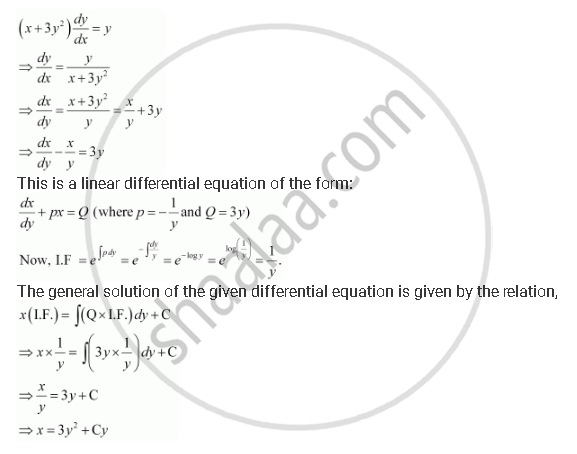Share

# For the Differential Equations Find the General Solution: (X + 3y^2) Dy/Dx = Y(Y > 0) - Mathematics

#### Question

For the differential equations find the general solution:

(x + 3y^2) dy/dx = y(y > 0)

#### SolutionIs there an error in this question or solution?
For the Differential Equations Find the General Solution: (X + 3y^2) Dy/Dx = Y(Y > 0) Concept: Methods of Solving First Order, First Degree Differential Equations - Linear Differential Equations.